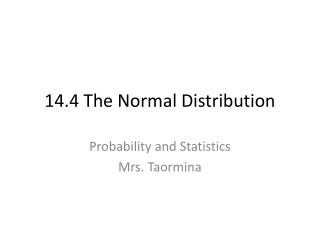DownloadDownload Presentation14.4 The Normal Distribution

# 14.4 The Normal Distribution

Download Presentation## 14.4 The Normal Distribution

- - - - - - - - - - - - - - - - - - - - - - - - - - - E N D - - - - - - - - - - - - - - - - - - - - - - - - - - -
##### Presentation Transcript

1. 14.4 The Normal Distribution Probability and Statistics Mrs. Taormina

2. Normal DistributionorNormal Curve • Most common distribution in real-life and in statistics • Curve Shaped • AKA: Bell-Curve

3. Properties of a Normal Distribution • A normal curve is bell shaped. • The highest point on the curve is at the mean of the distribution. (also median and mode) • The mean, median, and mode of the distribution are the same. • The total area under the curve is 1. • The 68-95-99.7 Rule

4. The 68-95-99.7 Rule • 68% of the data is within 1 standard deviation of the mean (highest point). This means there is 34% on each side. • 95% of the data is within 2 standard deviations of the mean. This means that there is 47.5% on each side. • 99.7% of the data is within 3 standard deviations of the mean. This means that there is 49.85% on each side.

5. The 68-95-99.7 Rule 34% 34% 2.35% 2.35% 13.5% 13.5% 68 % 95 % 99.7 % Nearly all (99.7%) of the values lie within 3 standard deviations of the mean (or between the mean minus 3 times the standard deviation and the mean plus 3 times the standard deviation).

6. EXAMPLE: Suppose a distribution of 1,000 scores represent scores on a standardized test. The mean of the distribution is 450 and the standard deviation is 25. How many scores do we expect to fall between 425 and 475? How many scores do we expect to fall above 500?

7. Part 1 If the mean is 450, 425 and 475 represent one standard deviation below and one standard deviation above the mean. This falls with 68% of the data. So .68 x 1000 = 680 So 680 scores are in the range of 425-475.

8. Part 2 500 represents 2 standard deviations above the mean (450 + 2(25)=500) 95% of our data falls within that range. We want to find the values above 500. This would be represented by (1/2) of 5% or 2.5% of the data. So, .025 x 1000 = 25 25 scores would be above 500.

9. Assignment: pg. 831 #5-16

10. Z-Scores • Represents the number of standard deviations a data value is from the mean. • Positive above mean, Negative below mean In the last example, we said that 500 was 2 standard deviations above (standard deviation was 25) the mean (which was 450). What would be the z-score? Z = 2

11. Z-Scores • The z-score table can be used for both positive and negative z-scores. • “A” represents the area underneath the curve. (Remember that the area underneath the entire curve is 1). • Area represents the percentage of scores within the specified range.

12. Finding Areas under a Normal Curve Using your z-score table, find the percentage of data (area under the curve) that lie in the following regions for a standard normal distribution: A). Between z = 0 and z = 1.3 B). Between z = 1.5 and z = 2.1 C). Between z = 0 and z = - 1.83

13. A). Between z = 0 and z = 1.3 By the z-score table, I look at z = 1.3 The area of the region is .403 This means 40.3% of the data will fall within this range.

14. B). Between z = 1.5 and z = 2.1

15. C). z = 0 and z = -1.83

16. Assignment Part 2 Pg. 831 #17-34

17. Converting Raw Scores to Z-Scores Value Mean Standard Deviation

18. Examples: Suppose the mean of a normal distribution is 20 and its standard deviation is 3. a). Find the z-score that corresponds to a raw score of 25. b). Find the z-score that corresponds to a raw score of 16. c). Find the raw score that corresponds to a z score of 2.1

19. Suppose the mean of a normal distribution is 20 and its standard deviation is 3.a). Find the z-score that corresponds to a raw score of 25.

20. Suppose the mean of a normal distribution is 20 and its standard deviation is 3.b). Find the z-score that corresponds to a raw score of 16.

21. Suppose the mean of a normal distribution is 20 and its standard deviation is 3.c). Find the raw score that corresponds to a z score of 2.1 +20 +20

22. ASSIGNMENT: Pg. 831 #35-72 (Due at the end of the hour on Friday along with your project!) Wednesday-Friday Meet in Media Center Bring in your collected data. I will need to check it in.